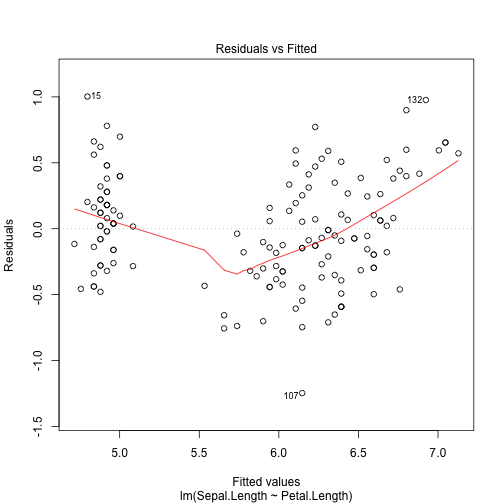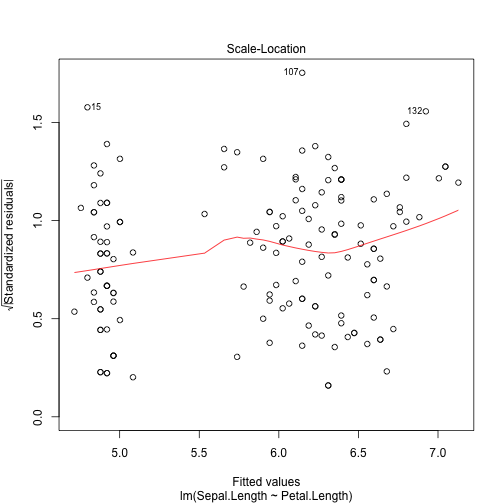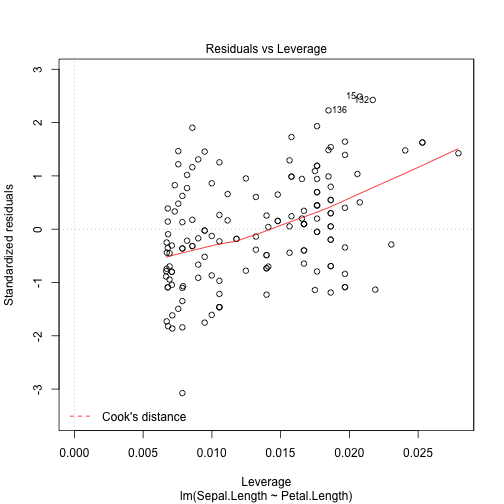# Introduction and linear models in R

Genomics and Systems Biology - 4/10/14 Author: Emily Davenport

## Description

In this class, you will get a brief introduction into the R programming environment and how to run linear models using this software. We'll start by using built in datasets, but then move on to an actual RNA-seq dataset that has been downloaded from GEO.

Learning objectives:

There are two goals for this lesson: 1. Be able to open R and load data in (this sounds easy but can be annoying sometimes!) 2. Have a sense of some of the built in functions you can use to look at your data (`head`, `table`, `summary`, etc) 3. Run linear models on data using R 3. Visualize results and validity of the model 4. Run many linear models on data using R

## Get familiar with R

R is a programming language designed for data analysis. In R, you can save data to 'variables'. You can then reuse these data just by calling the variable.

``````x <- 3

x
``````
``````##  3
``````

Variables can stand for more than one item. You can have a 'vector' of values saved to a variable:

``````y <- c(3, 10, 7, 9)
y
``````
``````##   3 10  7  9
``````

You can reference individual items in this vector by using its index. R is 1 based, so to reference the first item in the vector (the number 3) you would type this:

``````y
``````
``````##  3
``````

You can also store tables of data into a variable (either as a matrix or dataframe). You can refer to individual points of data by giving coordinates. In R, rows are referenced first, followed by columns.

``````mySweetTable <- matrix(c(3, 10, 7, 9, 6, 5, 1, 0), ncol = 2)
mySweetTable
``````
``````##      [,1] [,2]
## [1,]    3    6
## [2,]   10    5
## [3,]    7    1
## [4,]    9    0
``````

Let's say we want to look at the the value in row 1, column 2:

``````mySweetTable[1, 2]
``````
``````##  6
``````

Let's say I want to look at all of the values in row 1:

``````mySweetTable[1, ]
``````
``````##  3 6
``````

## Get familiar with the data

Today we are going to start with the iris dataset, which contains anatomic measurements and species classifications.

```````?`(iris)

dim(iris)
``````
``````##  150   5
``````
``````head(iris)
``````
``````##   Sepal.Length Sepal.Width Petal.Length Petal.Width Species
## 1          5.1         3.5          1.4         0.2  setosa
## 2          4.9         3.0          1.4         0.2  setosa
## 3          4.7         3.2          1.3         0.2  setosa
## 4          4.6         3.1          1.5         0.2  setosa
## 5          5.0         3.6          1.4         0.2  setosa
## 6          5.4         3.9          1.7         0.4  setosa
``````
``````
table(iris\$Species)
``````
``````##
##     setosa versicolor  virginica
##         50         50         50
``````

### Scatterplots

plot(x,y,…) # plots an x-y scatterplot

```````?`(plot)

plot(iris\$Petal.Length, iris\$Sepal.Length)
``````## Linear models in R (lm)

R has linear modelling capabilities. The function for running a linear model is:

lm(y ~ x,…)

y - response variable

x - covariates (can have as many as you want)

data - specifies the dataframe to be referenced

```````?`(lm)
``````

Is there a relationship between sepal length and petal length?

``````lm(Sepal.Length ~ Petal.Length, data = iris)
``````
``````##
## Call:
## lm(formula = Sepal.Length ~ Petal.Length, data = iris)
##
## Coefficients:
##  (Intercept)  Petal.Length
##        4.307         0.409
``````

As you can see, the lm() function returns you to the intercept and and beta estimation for the variable(s) you enter into your formula. Save this to a variable:

``````lm.out <- lm(Sepal.Length ~ Petal.Length, data = iris)
``````

If we print the variable, you can see that all of the information from the lm function is stored in that variable. We can now reference this later:

``````lm.out
``````
``````##
## Call:
## lm(formula = Sepal.Length ~ Petal.Length, data = iris)
##
## Coefficients:
##  (Intercept)  Petal.Length
##        4.307         0.409
``````

### How do I know if my covariates are significantly associated with my response variable?

Summarize (and perform statistics) on your linear model:

summary(object),…)

object - any object you want summarized (in our case, our lm.out variable)

```````?`(summary)
summary(lm.out)
``````
``````##
## Call:
## lm(formula = Sepal.Length ~ Petal.Length, data = iris)
##
## Residuals:
##     Min      1Q  Median      3Q     Max
## -1.2468 -0.2966 -0.0152  0.2768  1.0027
##
## Coefficients:
##              Estimate Std. Error t value Pr(>|t|)
## (Intercept)    4.3066     0.0784    54.9   <2e-16 ***
## Petal.Length   0.4089     0.0189    21.6   <2e-16 ***
## ---
## Signif. codes:  0 '***' 0.001 '**' 0.01 '*' 0.05 '.' 0.1 ' ' 1
##
## Residual standard error: 0.407 on 148 degrees of freedom
## Multiple R-squared:  0.76,   Adjusted R-squared:  0.758
## F-statistic:  469 on 1 and 148 DF,  p-value: <2e-16
``````
``````lm.out.sum <- summary(lm.out)
``````

You can see that the summary of our linear model has a lot of information. We can access all of this information piece by piece. R is an 'object-oriented' programming language. Each object in R has attributes that we can access using common names. To see the attributes of the summary(lm)-objects, you can type `names(lm.out.sum)`:

``````names(lm.out.sum)
``````
``````##   "call"          "terms"         "residuals"     "coefficients"
##   "aliased"       "sigma"         "df"            "r.squared"
##   "adj.r.squared" "fstatistic"    "cov.unscaled"
``````

If we just want to look at the estimated coefficients of our model we can type:

``````lm.out.sum\$coefficients
``````
``````##              Estimate Std. Error t value   Pr(>|t|)
## (Intercept)    4.3066    0.07839   54.94 2.427e-100
## Petal.Length   0.4089    0.01889   21.65  1.039e-47
``````

Pulling out the p-value (for some reason) is a bit harder. As you can see from the list of attributes, “pvalue” isn't one of them. We can, however, access the F-statistic that was calculated by the summary function:

``````lm.out.sum\$fstatistic
``````
``````## value numdf dendf
## 468.6   1.0 148.0
``````

Using the F statisic (value) and the degrees of freedom (numdf, dendf), we can calculated the pvalue:

```````?`(pf)

pvalue <- pf(lm.out.sum\$fstatistic, lm.out.sum\$fstatistic, lm.out.sum\$fstatistic,
lower.tail = FALSE)

pvalue
``````
``````##     value
## 1.039e-47
``````

### How do I know if my linear model meets assumptions?

Checking to see if your residuals are unbiased and have constant variance is easy in R. If you `plot` the lm function, you will see several summary graphs of your linear model:

``````plot(lm.out)
``````You could also do this by hand if you wanted. You can access the residuals (lm.out\$residuals) and the fitted values (lm.out\$fitted.values) in your model and plot them using a scatter plot.

### How can I plot the best fit line on my graph?

If you want to plot a trendline of your data, you can access the coefficients attribute of your linear model, and use those as terms in a trendline.

abline()

a - intercept

b - slope

h - y-value for horizontal line

v - x-value for vertical line

``````plot(iris\$Petal.Length, iris\$Sepal.Length)
abline(a = lm.out.sum\$coefficients, b = lm.out.sum\$coefficients)
``````## Linear models on real genomic data

Let's load some real genomic data and start to play with it. The data for this lesson can be found at the following url: http://www.ncbi.nlm.nih.gov/geo/query/acc.cgi?acc=GSE45263

Scroll to the bottom of the page and you'll see a file called: GSE45263_gene.expression.measurements.txt.gz

Download that text file and move it to your desktop.

In RStudio, go to the Session menu at the top -> Set Working Directory -> Choose Directory and set that to your desktop (or wherever you saved the file from the internet)

To load data into R, you can use the `read.table()` function

read.table(file, …)

file - set file=“yourdata.txt”

header - set to TRUE if the first line of your file describes the data (versus is data)

sep - set the delimiter between the entries

Let's load our data:

``````mydata <- read.table(file = "GSE45263_gene.expression.measurements.txt.gz",
header = TRUE, sep = "\t")
``````

Note that R will take gzipped files and unpack them for you into R. This means you don't have to unzip huge data files when you want to use them and save space on your computer.

Let's take a look at our data:

``````dim(mydata)
``````
``````##  25813    21
``````
``````head(mydata)
``````
``````##            ID_REF Human_AF_8 Human_EU_11 Human_EU_4 Human_AF_12
## 1 ENSG00000000003     0.1394    -0.27962    -0.6147     -0.6155
## 2 ENSG00000000005    -0.8168    -0.81676     0.5224     -0.8168
## 3 ENSG00000000419     0.4391     2.83123    -0.2066     -0.9811
## 4 ENSG00000000457    -1.4025    -0.07813    -0.6768     -0.0549
## 5 ENSG00000000460    -0.8636    -0.02775    -1.0508     -0.2800
## 6 ENSG00000000938     1.7407     0.51451    -0.8887     -0.7476
##   Human_AS_13 Human_AS_7 Human_AS_9 Human_AS_5 Human_AS_10 Human_EU_14
## 1    -0.07937    -0.5277   -0.74929  -0.783248     -0.3219     0.26156
## 2    -0.35667     0.3393    0.93672  -0.000234     -0.8168     0.04095
## 3     0.04231    -0.3811    0.20583  -0.737685     -1.0845     0.52695
## 4    -0.71318    -0.9412   -0.38722  -0.901445     -0.6500    -0.13818
## 5    -0.40892    -0.6287   -0.09292  -0.256085     -0.4954    -0.24508
## 6     0.15036     2.7379   -0.77159  -0.956635     -0.4133     1.13971
##   Human_EU_3 Human_AF_2 Human_AF_6 Human_EU_1 Chimpanzee_4 Chimpanzee_6
## 1    -0.7471     3.0816     2.3511    -0.4473      -0.1858      0.19963
## 2     1.7703    -0.8168    -0.3370     1.3984       2.6947     -0.59724
## 3    -0.3840    -1.1009    -1.1716    -0.5400       0.7904     -0.26222
## 4     0.9170     1.5282    -0.9481    -0.4474       1.9200     -0.18687
## 5     2.6386     2.3789    -0.7781    -0.3478       0.9998     -0.01414
## 6    -0.2126    -0.2695     1.2141    -0.2226      -1.1292     -0.29129
##   Chimpanzee_5 Chimpanzee_1 Chimpanzee_3 Chimpanzee_2
## 1       0.4291      -0.5134     -0.42309      -0.1745
## 2      -0.7190      -0.4679     -0.74670      -0.3941
## 3      -0.5113       0.8988     -0.04969       1.6762
## 4       1.4538      -0.7658      1.15496       1.3178
## 5      -0.2064      -0.8131      0.93731      -0.4459
## 6      -0.4345      -0.6202     -0.00830      -0.5314
``````

Let's make that first column (which are gene names) the rownames, rather than as part of our table:

``````rownames(mydata) <- mydata\$ID_REF
mydata\$ID_REF <- NULL
mydata <- as.matrix(mydata)
``````

In this dataset we have human and chimp data. Let's make a variable that indicates whether a sample is human or chimp:

``````species <- c(rep("human", 14), rep("chimp", 6))
species
``````
``````##   "human" "human" "human" "human" "human" "human" "human" "human"
##   "human" "human" "human" "human" "human" "human" "chimp" "chimp"
##  "chimp" "chimp" "chimp" "chimp"
``````

Are there differences in gene expression between humans and chimps? Let's start with just the one gene.

Let's make a boxplot to see visually if there are differences between the chimps and the humans:

``````boxplot(mydata[1, ] ~ species)
``````Meh? Can you tell by eyeballing if there's a significant difference between chimp and human? Let's make a linear model where we have gene expression as our dependent variable and species (as a dummy variable) for our independent variable.

Y ~ beta0 + beta1X + E

Y = dependent variable (gene expression) beta0 = mean expression level in chimps beta1 = the increase (or decrease) of gene expression on average in the subject is human (1 for human, 0 if chimp) E = error

Note: the order of your variables will set what the intercept is. We have humans listed first in our dataset, so the model will have the dummy variable be 1 if human, 0 if chimp. If we had chimps listed first, it would have 1 if chimp, 0 if human.

``````apelm <- lm(mydata[1, ] ~ species)
apelm.sum <- summary(apelm)
apelm.sum
``````
``````##
## Call:
## lm(formula = mydata[1, ] ~ species)
##
## Residuals:
##    Min     1Q Median     3Q    Max
## -0.831 -0.597 -0.320  0.122  3.034
##
## Coefficients:
##              Estimate Std. Error t value Pr(>|t|)
## (Intercept)    -0.111      0.418   -0.27     0.79
## specieshuman    0.159      0.500    0.32     0.75
##
## Residual standard error: 1.02 on 18 degrees of freedom
## Multiple R-squared:  0.00559,    Adjusted R-squared:  -0.0497
## F-statistic: 0.101 on 1 and 18 DF,  p-value: 0.754
``````

Ok, the first gene isn't significant, but let's test all genes:

``````mypvals <- c()
for (i in 1:dim(mydata)) {
apelm.sum <- summary(lm(mydata[i, ] ~ species))
pval <- pf(apelm.sum\$fstatistic, apelm.sum\$fstatistic, apelm.sum\$fstatistic,
lower.tail = FALSE)
mypvals <- c(mypvals, pval)
}
``````

How many genes did we just test?

``````length(mypvals)
``````
``````##  25813
``````
``````dim(mydata)
``````
``````##  25813    20
``````

What is our lowest p-value?

``````min(mypvals)
``````
``````##  4.869e-24
``````

What does our distribution of p-values look like?

``````hist(mypvals)
``````How many p-values are lower than a Bonferroni threshold (p < 0.05)?

``````thresh <- 0.05/length(mypvals)
thresh
``````
``````##  1.937e-06
``````
``````length(which(mypvals < thresh))
``````
``````##  1399
``````

What about if we correct using FDR?

```````?`(p.adjust)
FDR.adjusted.pvals <- p.adjust(mypvals, method = "BH")
length(which(FDR.adjusted.pvals < 0.05))
``````
``````##  8743
``````

How can I calculate q-values?

First, install and then load the library:

NOTE: Save your markdown file now if you've made changes you don't want to lose. When I upgraded to Mavericks, I would get an error that would shut down R every time I tried to load the qvalues package. It sucked and I don't want you to lose your notes!

``````source("http://bioconductor.org/biocLite.R")
``````
``````## Bioconductor version 2.12 (BiocInstaller 1.10.4), ?biocLite for help
## A newer version of Bioconductor is available for this version of R,
##   ?BiocUpgrade for help
``````
``````biocLite("qvalue")
``````
``````## BioC_mirror: http://bioconductor.org
## Using Bioconductor version 2.12 (BiocInstaller 1.10.4), R version 3.0.2.
## Installing package(s) 'qvalue'
``````
``````##
## The downloaded binary packages are in
##  /var/folders/dr/3dgq3bh965q09704nz36lp9r0000gn/T//Rtmps1NQ4J/downloaded_packages
``````
``````library(qvalue)
``````

Calculate qvalues:

```````?`(qvalue)
myqvalues <- qvalue(mypvals)
names(myqvalues)
``````
``````##  "call"    "pi0"     "qvalues" "pvalues" "lambda"
``````
``````myqvals <- myqvalues\$qvalues
length(which(myqvals < 0.05))
``````
``````##  11625
``````

## Multiple regression

Ok, let's say in our experiment we want to model some additional information about just the human samples, for instance, what population they belong to, how old the individuals are, and their sex.

Let's pull out just the human samples:

``````humans <- mydata[, grep("Human", colnames(mydata))]
``````

We need to generate the table of covariate information. The population information is encoded in the names of those samples, but age and sex aren't given to us (for this exercise I'm just going to make up this data, but clearly you shouldn't just make up covariates for your real data!)

``````age <- c(20.2, 19.1, 18.5, 18.5, 20.1, 19, 18.7, 18.6, 18.5, 21.2, 18.8, 30.1,
27.4, 19)
sex <- c(rep("male", 7), rep("female", 7))
pop <- c()
for (i in 1:length(colnames(humans))) {
if (grepl("EU", colnames(humans)[i])) {
pop <- c(pop, "EUR")
} else if (grepl("AF", colnames(humans)[i])) {
pop <- c(pop, "AFR")
} else {
pop <- c(pop, "ASN")
}
}
``````

Ok, let's see for one gene if any of these covariates are associated with gene expression:

``````mylm <- lm(humans[549, ] ~ age + sex + pop)
summary(mylm)
``````
``````##
## Call:
## lm(formula = humans[549, ] ~ age + sex + pop)
##
## Residuals:
##     Min      1Q  Median      3Q     Max
## -1.4193 -0.3608 -0.0792  0.1058  1.7326
##
## Coefficients:
##             Estimate Std. Error t value Pr(>|t|)
## (Intercept)  -4.8434     2.6870   -1.80    0.105
## age           0.2405     0.1037    2.32    0.045 *
## sexmale      -0.2748     0.5617   -0.49    0.636
## popASN        0.0344     0.7751    0.04    0.966
## popEUR        0.5492     0.7902    0.70    0.505
## ---
## Signif. codes:  0 '***' 0.001 '**' 0.01 '*' 0.05 '.' 0.1 ' ' 1
##
## Residual standard error: 0.885 on 9 degrees of freedom
## Multiple R-squared:  0.601,  Adjusted R-squared:  0.424
## F-statistic: 3.39 on 4 and 9 DF,  p-value: 0.0594
``````

Hm, so, our model isn't significant on the whole, but age looks like it might be significant. We have sex and population also in our model and they don't look like they're associated. Let's look at a model with just age included:

``````summary(lm(humans[549, ] ~ age))
``````
``````##
## Call:
## lm(formula = humans[549, ] ~ age)
##
## Residuals:
##     Min      1Q  Median      3Q     Max
## -1.4510 -0.2458 -0.1060  0.0024  2.2145
##
## Coefficients:
##             Estimate Std. Error t value Pr(>|t|)
## (Intercept)  -4.6543     1.3384   -3.48   0.0046 **
## age           0.2348     0.0642    3.66   0.0033 **
## ---
## Signif. codes:  0 '***' 0.001 '**' 0.01 '*' 0.05 '.' 0.1 ' ' 1
##
## Residual standard error: 0.834 on 12 degrees of freedom
## Multiple R-squared:  0.527,  Adjusted R-squared:  0.487
## F-statistic: 13.4 on 1 and 12 DF,  p-value: 0.00329
``````

This was a case where we were including too many variables in the model. Sex and population were included, but didn't actually explain much of the variation in gene expression. By having them in the model, we increased the degrees of freedom, making it more difficult to reach significance.

### Problems:

a) Write a linear model to to test if gene expression for the first gene in the table is associated with the three populations in this study. What do each of the terms in the model stand for?

b) How many variables are included in the model?

c) What is the interpretation of the intercept in the model? What is the interpretation of the betas that you're estimating?

d) How many genes in this dataset are associated with population with a p-value < 0.05?

e) How many of those are significant after a Bonferroni correction?

f) What about controlling for FDR at alpha = 0.05?

a) Write a linear model to to test if gene expression for the first gene in the table is associated with the three populations in this study and age. What do each of the terms in the model stand for?

b) How many variables are included in the model?

c) What is the interpretation of the intercept in the model? What is the interpretation of the betas that you're estimating?

d) How many genes in this dataset are associated with population and age with a p-value < 0.05?

e) How many of those are significant after a Bonferroni correction?

f) What about controlling for FDR at alpha = 0.05?